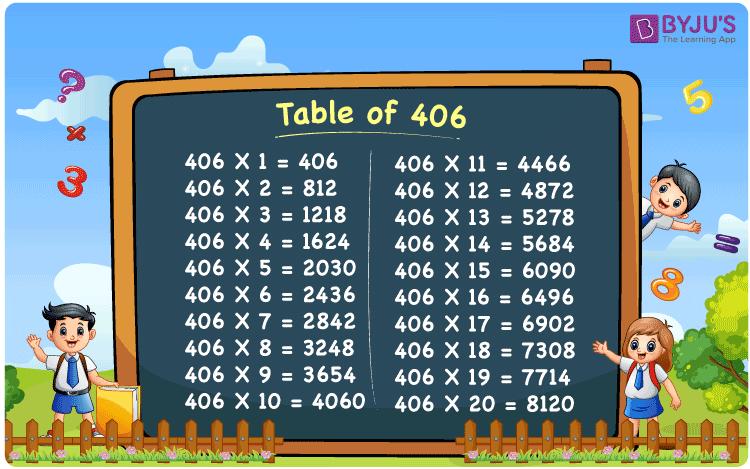Checkout JEE MAINS 2022 Question Paper Analysis : Checkout JEE MAINS 2022 Question Paper Analysis :

# Table of 406

The Table of 406 piques pupils’ curiosity in learning Mathematics. Learning multiplication tables improves exam skills by making it easier to solve complicated problems. Students can download the table in PDF version from BYJU’S for free, depending on their needs.

## Table of 406 Chart## What is the 406 Times Table?

The Table of 406 is a 406 multiplication table that shows the value when the number 406 is multiplied or added to another whole number. For speedier calculations, pupils should memorise the table up to ten values. Additionally, students are encouraged to download the table in PDF version whenever necessary to aid with their mathematics studies. For the first ten numbers from the chart below, go through the values of table 406.

 406×1 = 406 406 406×2 = 812 406 + 406 = 812 406×3 = 1218 406 + 406 + 406 = 1218 406×4 = 1624 406 + 406 + 406 + 406 = 1624 406×5 = 2030 406 + 406 + 406 + 406 + 406 = 2030 406×6 = 2436 406 + 406 + 406 + 406 + 406 + 406 = 2436 406×7 = 2842 406 + 406 + 406 + 406 + 406 + 406 + 406 = 2842 406×8 = 3248 406 + 406 + 406 + 406 + 406 + 406 + 406 + 406 = 3248 406×9 = 3654 406 + 406 + 406 + 406 + 406 + 406 + 406 + 406 + 406 = 3654 406×10 = 4060 406 + 406 + 406 + 406 + 406 + 406 + 406 + 406 + 406 + 406 = 4060

## Multiplication Table of 406

The multiplication table of 406 is given below in the tabular form:

 406 × 1 = 406 406 × 2 = 812 406 × 3 = 1218 406 × 4 = 1624 406 × 5 = 2030 406 × 6 = 2436 406 × 7 = 2842 406 × 8 = 3248 406 × 9 = 3654 406 × 10 = 4060 406 × 11 = 4466 406 × 12 = 4872 406 × 13 = 5278 406 × 14 = 5684 406 × 15 = 6090 406 × 16 = 6496 406 × 17 = 6902 406 × 18 = 7308 406 × 19 = 7714 406 × 20 = 8120

## Solved Example on Table of 406

1: The cost of one coconut candy is Rs 20. Find the value of 406 coconut candies.

Solution:

The cost of one coconut candy = Rs 20

Cost of 286 coconut candies = 20 x 406 = 8120

Therefore, the value of 406 coconut candies is Rs 8120

## Frequently Asked Questions on the Table of 406

### What is the table of 406?

The table of 406 is the repeated addition of 406 for a number of times. The table of 406 is also known as the 406 times table.

### What is 406 times 2?

406 times 2 is 812. I.e., 406 × 2 = 812.

### Determine the value 406 times 20 minus 120.

406 times 20 minus 120 equals 5000.
I.e., 406 × 20 – 120 = 8000.